• 考研数学公式，三角函数公式（倍角公式，三角函数求导常用公式，倍角公式 三倍角公式 ，半角公式，和差化积，积化和差 ）
• 高中数学三角函数公式轻松记：正弦余弦的两角和差公式“口诀”记上面是介绍的正弦和余弦的两角和差公式如何熟记，主要是应用口诀“正异同，余同异”快速掌握。高中数学三角函数公式轻松记：正切余切两角和差公式的...
在掌握了三角函数两角和差公式之后，我们可以根据两角和差公式，轻易地掌握三角函数倍角公式和半角公式。如果还没有掌握两角和差公式，可以先参看相关的内容，待掌握后再进行下面的环节，否则效果不佳。高中数学三角函数公式轻松记：正弦余弦的两角和差公式“口诀”记上面是介绍的正弦和余弦的两角和差公式如何熟记，主要是应用口诀“正异同，余同异”快速掌握。高中数学三角函数公式轻松记：正切余切两角和差公式的推导与记忆上面是介绍正切和余切的两角和差公式如何推导及快速记忆之法。在这些熟练掌握后，我们就能很轻易地掌握和运用倍角公式和半角公式了。三角函数二倍角公式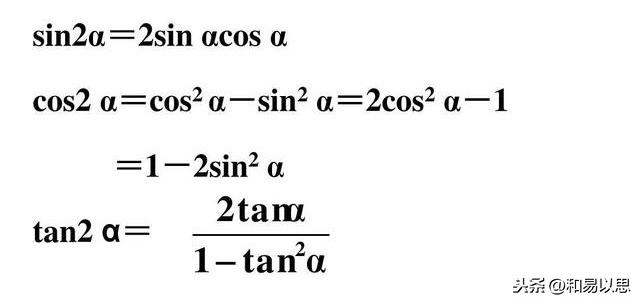三角函数二倍角公式在三角函数加法公式(即两角和差公式)中我们学习的是有两个角，其中一个用α表示，另一个用β表示。当我们现在用来记二倍角公式时，也就是一个角的2倍，而一个角的两倍就是这个角和自己相加的结果。所以我们把两角和差公式中的两个不同角变为相同的角时，两角和差公式也就成了二倍角公式。比如sin(α+β)当α=β时，sin(α+β)=sin2α=sin2β，后者不就是二倍角吗？所以只要掌握了三角函数的两角和差公式，我们把公式中的不同的两个角当作相同的角时就直接可以写出二倍角公式了。sin2α=sin(α+α)=sinαcosα+cosαsinα=2sinαcosα.同理可以写出其他形式的三角函数的二倍角公式，大家不妨自己写一下看看。这里要提示下的是余弦的二倍角公式在写出后，然后利用sinα+cosα=1这个关系式，又可以推导出两个公式。比如cos2α=cos(α＋α)根据口诀“余同异”，可以直接写出余弦的两角和的公式如下：cos2α=cos(α＋α)=cosαcosα-sinαsinα=cos²α-sin²α.①根据sin²α+cos²α=1，可以分别得出 sin²α=1-cos²α和cos²α=1-sin²α。分别代入①式就可以得出余弦的二倍角公式的另外两个常用的公式表达式了。二倍角公式上面是推理来的，如果不推理，也可以利用前面介绍的方法通过观察找特点直接写出公式。比如sin2α,这是正弦，按照“正异同”知道其公式展开式中的每一项都是相异的同组组成的，所以每一项都是sinαcosα,这样看到正弦可以直接写出此项，然后前面加个2就可以了。(想想为什么)再看cos2α,根据口诀“余同异”，可以直接写出cos²α-sin²α.三角函数半角公式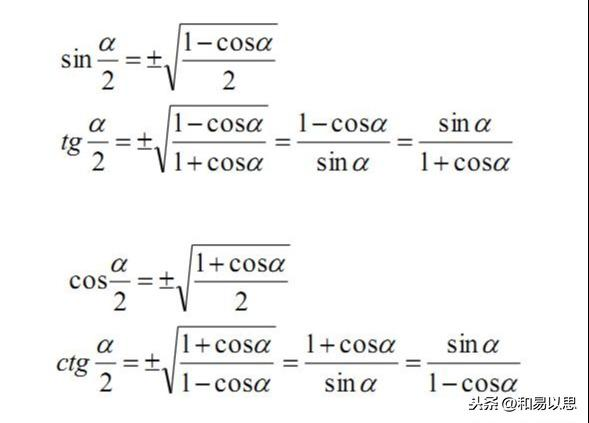三角函数半角公式这里的tg也就是tan,是正切；ctg也就是cot,是余切。不过现在教材更多采用的是tan和cot来表示正切和余切。可能大家一看公式，感觉很复杂，不好记。其实这些公式都是来自于余弦的两角和差公式。余弦的两角和差公式，当我们把两个不同的角当作相同的角看待时就变成了余弦的二倍角公式。其中一个是：cos2α=2cos²α-1 ②另一个是： cos2α=1-2sin²α ③为何采用余弦的二倍角公式而不是正弦的二倍角公式？原因就在于正弦的二倍角公式等式右面不知一个三角函数，而是同组的相异的两个三角函数。我们现在观察②式，发现等号左边的角是 2α，等号右边的角是 α，有没有发现什么特点？右面的角是左边的角的一半！③式也同样如此。也就是说等式左边的角是右边的2倍，所以是二倍角公式。从右边的角的角度来看，右边的角是左边的角的一半，岂不就是半角公式吗？当然根据公式的常用表示法，一般展开式在右边，所以我们需要把半角放在等式的左边。由此有：②式变换为 2cos²α-1 =cos2α → 2cos²α=1+cos2α → cos²α=(1+cos2α)/2,然后开方就可以得到公式的形式。(切记：开平方结果有两个，一正一负，具体选择哪个符号，还是取决半角函数的符号)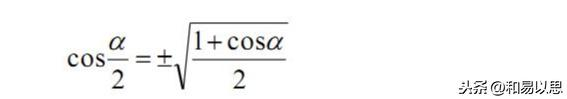余弦半角公式为了符合我们一般的习惯，我们不用α表示半角，而是用α/2表示，所以2α也对应变成了α。所以余弦的二倍角公式，因而变为：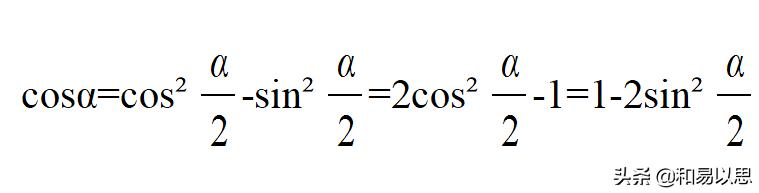根据这个式子可以得出三角函数的降幂公式，只需要再变化下即可。这个不再展开论述。这个方法也就是比较等式两边的角的关系，然后由一边的角表示另一边，这样就可以得到不同的公式，不过从根源上看还都是一个公式的变化而已。如果没有掌握这个特点，很可能会为公式繁多而忧虑，并且还很容易遗忘或记混淆。思路：余弦的两角和差公式 → 二倍角公式(令两角相等)→ 半角公式(比较等式两边角的关系) → 降幂公式。如：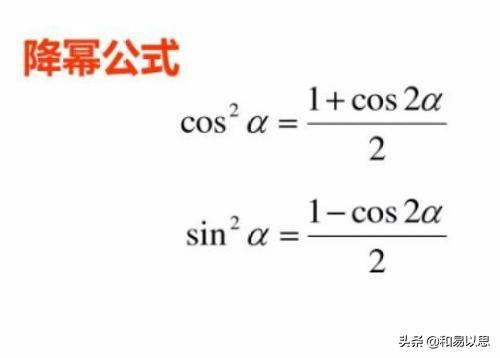三角函数降幂公式由于这里是介绍公式记忆和方法的，所以不再论述不同公式如何使用以及在具体题目中我们应该如何根据题目条件和要求确定用哪个公式。正切和余切的半角公式，也是先用正弦除以余弦公式，然后将对应的正弦和余弦的半角公式代入，最后采用分子有理化或分母有理化而分别得到两个去除根式的公式表达形式。(无论是根式中分子或分母中的1+cosα还是1-cosα,都是采用构成1-cosα的形式进行分母或分子有理化的，因为这样就是sinα,可以直接开出来了。这也是平方差公式的逆运用。)
展开全文• 半角公式（Half angle formula）是利用某个角（如∠A）的正弦值、余弦值、正切值，及其他三角函数值，来求其半角的正弦值，余弦值，正切值，及其他三角函数值的公式公式 常用的半角公式包括以下三个： 半角...
半角公式

半角公式（Half angle formula）是利用某个角（如∠A）的正弦值、余弦值、正切值，及其他三角函数值，来求其半角的正弦值，余弦值，正切值，及其他三角函数值的公式。

公式

常用的半角公式包括以下三个：

半角正弦公式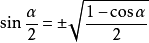半角余弦公式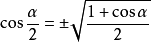半角正切公式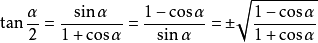以上三角函数值的正负由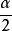所在的象限决定。

公式推导

和角公式推导：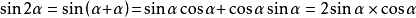半角正弦公式

由上述等式cos2α，整理得：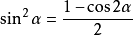将代入α，整理得：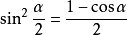开方，得半角余弦公式

由等式①整理得：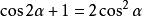将代入，整理得：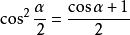开方，得半角正切公式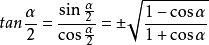展开全文• 三角函数公式 两角和公式 倍角公式 三倍角公式 半角公式 和差化积 积化和差 诱导公式 万能公式 其它公式 其他非重点三角函数 双曲函数 公式一 ...


初等函数的图形

幂函数的图形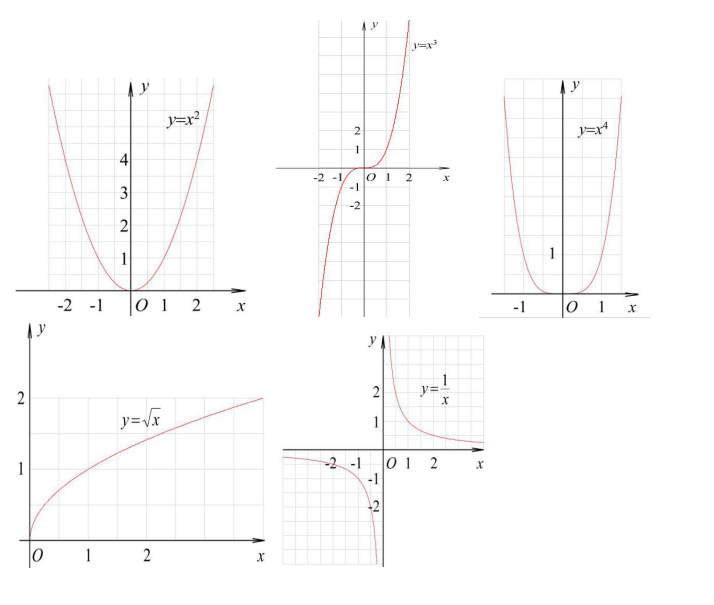指数函数的图形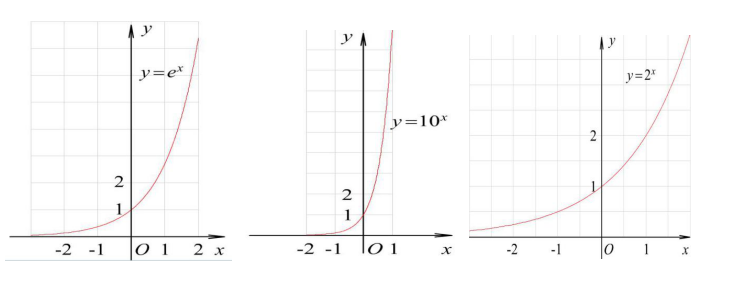对数函数的图形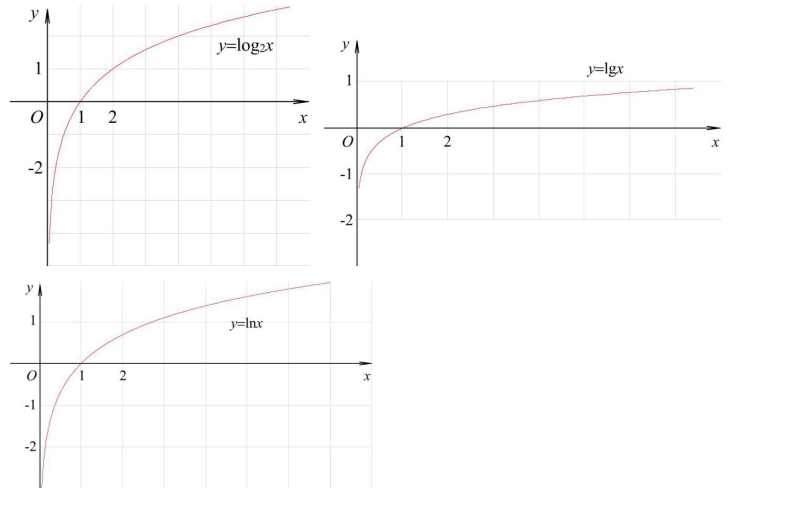三角函数的图形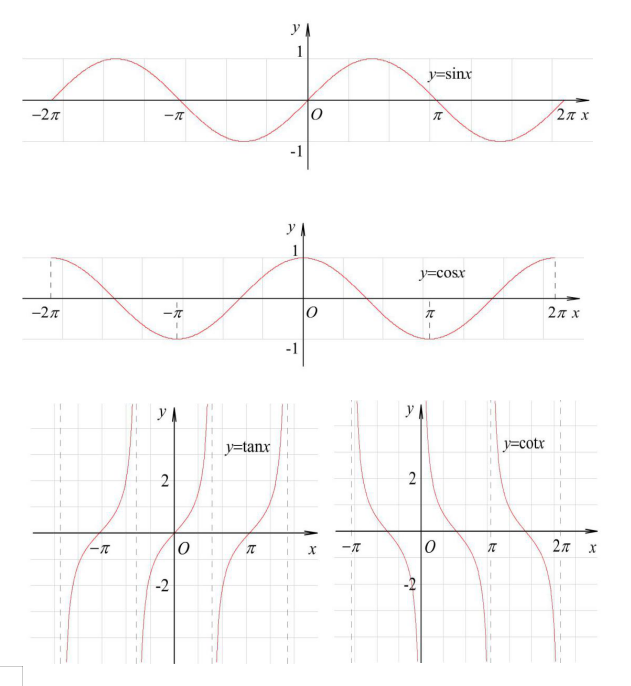反三角函数的图形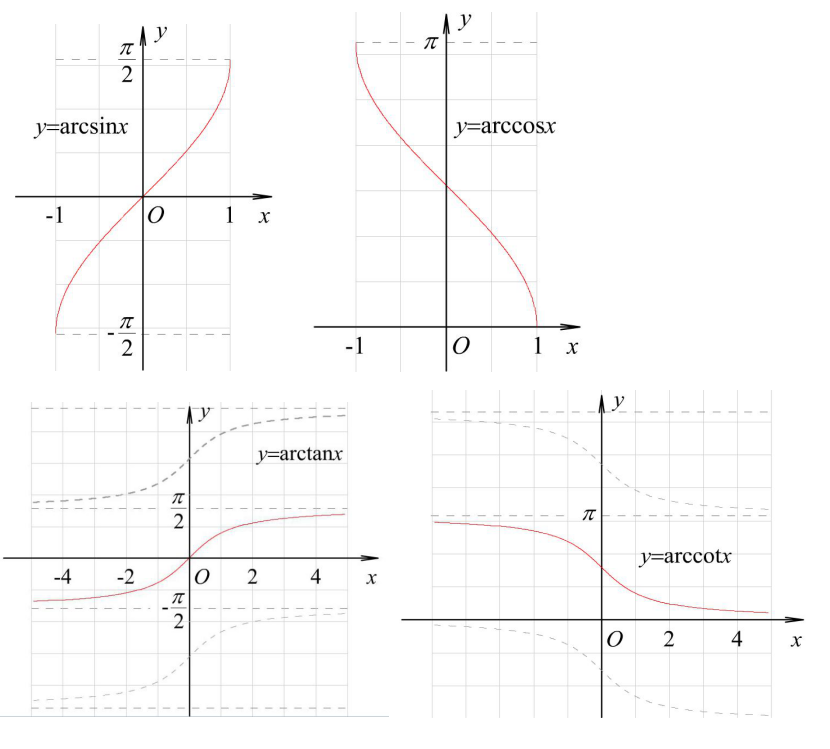各三角函数值在各象限的符号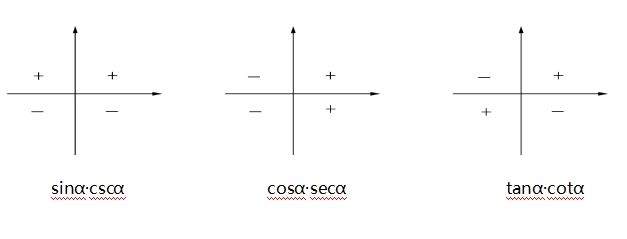三角函数的性质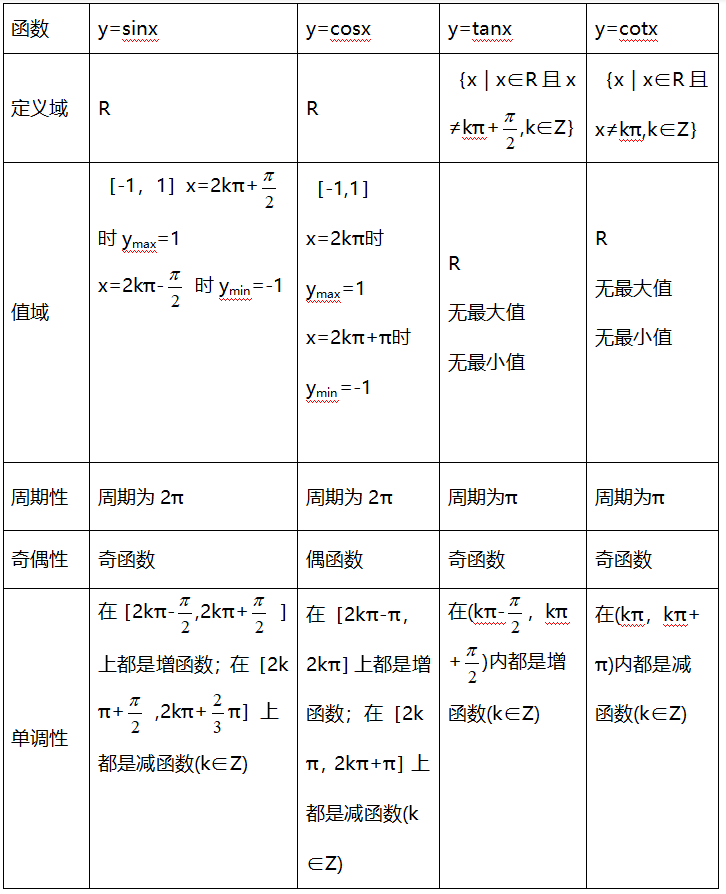反三角函数的性质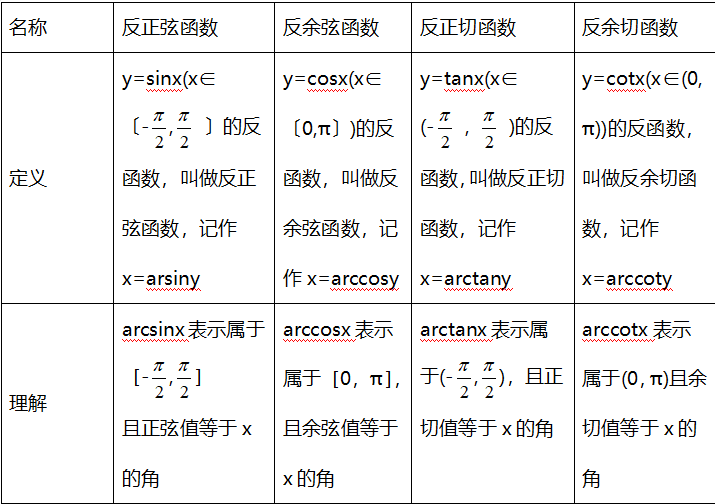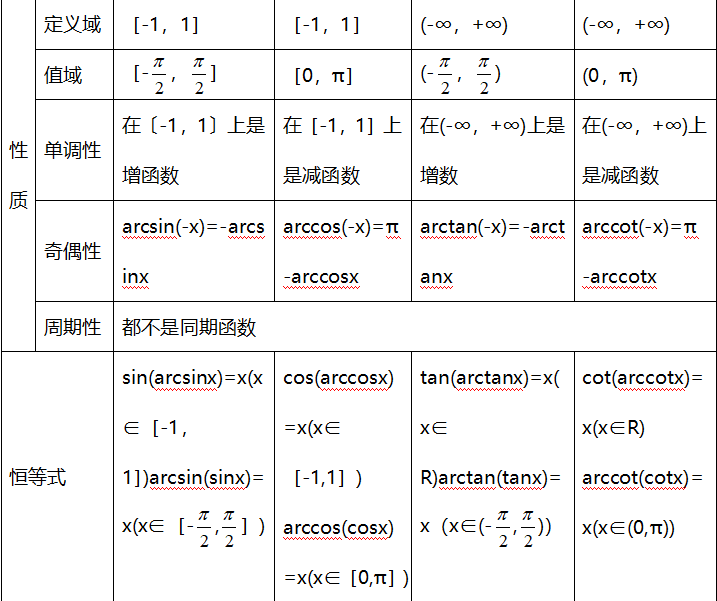三角函数公式

两角和公式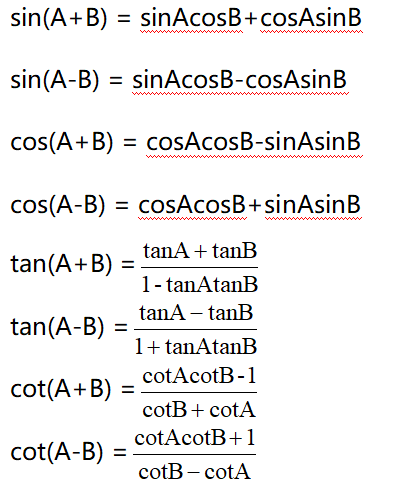倍角公式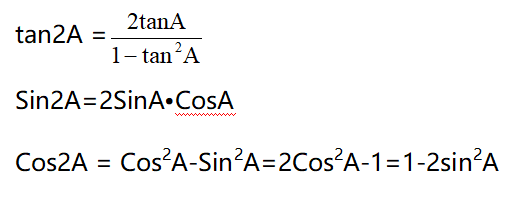三倍角公式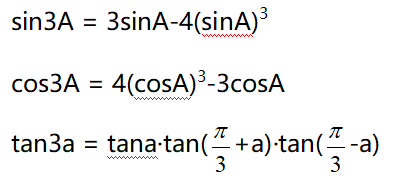半角公式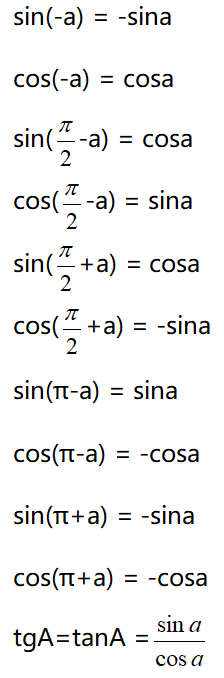和差化积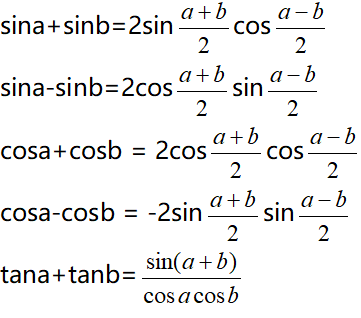积化和差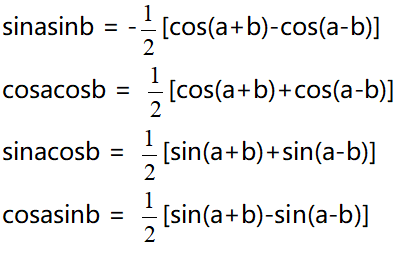诱导公式万能公式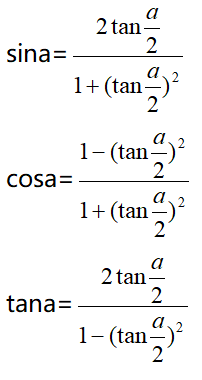其它公式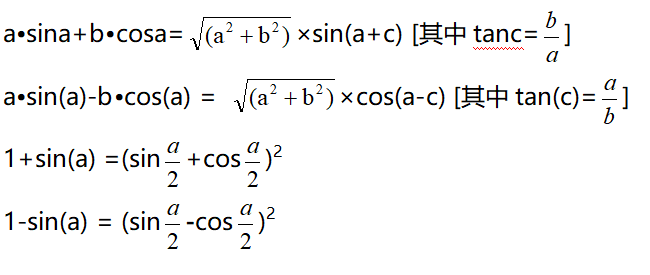其他非重点三角函数双曲函数公式一

设α为任意角，终边相同的角的同一三角函数的值相等：

sin（2kπ＋α）= sinα

cos（2kπ＋α）= cosα

tan（2kπ＋α）= tanα

cot（2kπ＋α）= cotα

公式二

设α为任意角，π+α的三角函数值与α的三角函数值之间的关系：

sin（π＋α）= -sinα

cos（π＋α）= -cosα

tan（π＋α）= tanα

cot（π＋α）= cotα

公式三

任意角α与 -α的三角函数值之间的关系：

sin（-α）= -sinα

cos（-α）= cosα

tan（-α）= -tanα

cot（-α）= -cotα

公式四

利用公式二和公式三可以得到π-α与α的三角函数值之间的关系：

sin（π-α）= sinα

cos（π-α）= -cosα

tan（π-α）= -tanα

cot（π-α）= -cotα

公式五

利用公式-和公式三可以得到2π-α与α的三角函数值之间的关系：

sin（2π-α）= -sinα

cos（2π-α）= cosα

tan（2π-α）= -tanα

cot（2π-α）= -cotα

公式六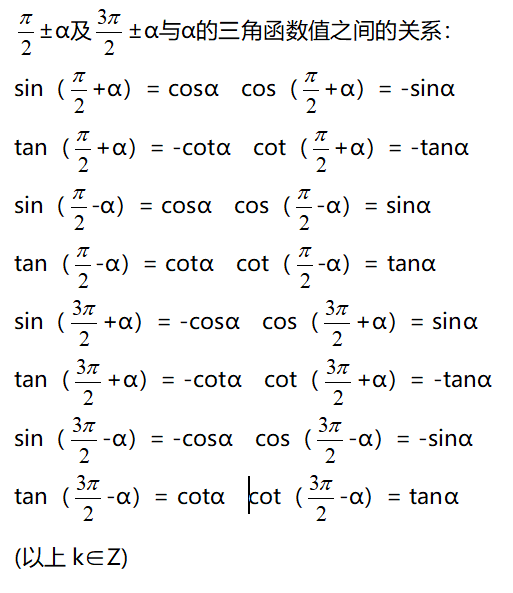这个物理常用公式我费了半天的劲才输进来,希望对大家有用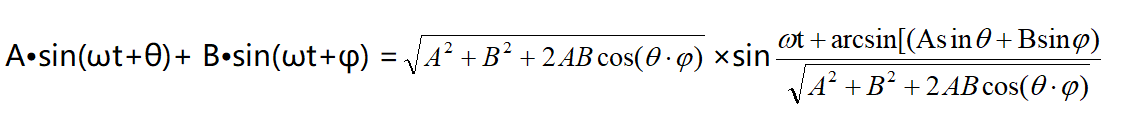三角函数公式证明（全部）

公式表达式

乘法与因式分解

a2-b2=(a+b)(a-b) a3+b3=(a+b)(a2-ab+b2) a3-b3=(a-b)(a2+ab+b2)

三角不等式

|a+b|≤|a|+|b|

|a-b|≤|a|+|b|

|a|≤b<=>-b≤a≤b

|a-b|≥|a|-|b|

-|a|≤a≤|a|

一元二次方程的解

-b+√(b2-4ac)/2a -b-b+√(b2-4ac)/2a

根与系数的关系

X1+X2=-b/a

X1*X2=c/a

注：韦达定理

判别式 b2-4a=0 注：方程有相等的两实根

b2-4ac>0 注：方程有一个实根

b2-4ac<0 注：方程有共轭复数根

三角函数公式

两角和公式

sin(A+B)=sinAcosB+cosAsinB sin(A-B)=sinAcosB-sinBcosA

cos(A+B)=cosAcosB-sinAsinB cos(A-B)=cosAcosB+sinAsinB

tan(A+B)=(tanA+tanB)/(1-tanAtanB) tan(A-B)=(tanA-tanB)/(1+tanAtanB)

ctg(A+B)=(ctgActgB-1)/(ctgB+ctgA) ctg(A-B)=(ctgActgB+1)/(ctgB-ctgA)

倍角公式

tan2A=2tanA/(1-tan2A) ctg2A=(ctg2A-1)/2ctga

cos2a=cos2a-sin2a=2cos2a-1=1-2sin2a

半角公式

sin(A/2)=√((1-cosA)/2) sin(A/2)=-√((1-cosA)/2)

cos(A/2)=√((1+cosA)/2) cos(A/2)=-√((1+cosA)/2)

tan(A/2)=√((1-cosA)/((1+cosA)) tan(A/2)=-√((1-cosA)/((1+cosA))

ctg(A/2)=√((1+cosA)/((1-cosA)) ctg(A/2)=-√((1+cosA)/((1-cosA))

和差化积

2sinAcosB=sin(A+B)+sin(A-B) 2cosAsinB=sin(A+B)-sin(A-B)

2cosAcosB=cos(A+B)-sin(A-B) -2sinAsinB=cos(A+B)-cos(A-B)

sinA+sinB=2sin((A+B)/2)cos((A-B)/2 cosA+cosB=2cos((A+B)/2)sin((A-B)/2)

tanA+tanB=sin(A+B)/cosAcosB tanA-tanB=sin(A-B)/cosAcosB

ctgA+ctgBsin(A+B)/sinAsinB -ctgA+ctgBsin(A+B)/sinAsinB

某些数列前n项和

1+2+3+4+5+6+7+8+9+…+n=n(n+1)/2

1+3+5+7+9+11+13+15+…+(2n-1)=n2

2+4+6+8+10+12+14+…+(2n)=n(n+1)

12+22+32+42+52+62+72+82+…+n2=n(n+1)(2n+1)/6

13+23+33+43+53+63+…n3=n2(n+1)2/4

1*2+2*3+3*4+4*5+5*6+6*7+…+n(n+1)=n(n+1)(n+2)/3

正弦定理

a/sinA=b/sinB=c/sinC=2R

注： 其中 R 表示三角形的外接圆半径

余弦定理

b2=a2+c2-2accosB

注：角B是边a和边c的夹角

正切定理

[(a+b)/(a-b)]={[Tan(a+b)/2]/[Tan(a-b)/2]}

圆的标准方程

(x-a)2+(y-b)2=r2 注：（a,b）是圆心坐标

圆的一般方程

x2+y2+Dx+Ey+F=0 注：D2+E2-4F>0

抛物线标准方程

y2=2px y2=-2px x2=2py x2=-2py

直棱柱侧面积

S=c*h

斜棱柱侧面积

S=c'*h

正棱锥侧面积

S=1/2c*h'

正棱台侧面积

S=1/2(c+c')h'

圆台侧面积

S=1/2(c+c')l=pi(R+r)l

球的表面积

S=4pi*r2

圆柱侧面积

S=c*h=2pi*h

圆锥侧面积

S=1/2*c*l=pi*r*l

弧长公式

l=a*r

a是圆心角的弧度数r >0

扇形面积公式

s=1/2*l*r

锥体体积公式

V=1/3*S*H

圆锥体体积公式

V=1/3*pi*r2h

斜棱柱体积

V=S'L

注：其中,S'是直截面面积， L是侧棱长

柱体体积公式

V=s*h

圆柱体

V=pi*r2h


展开全文• 一、三角函数定义 二、诱导公式 三、倒数关系 四、平方关系 五、两角和与差的三角函数 六、积化和差公式 七、和差化积公式 八、倍角公式 九、半角公式 十、万能公式 十一、其他公式 ...
一、三角函数定义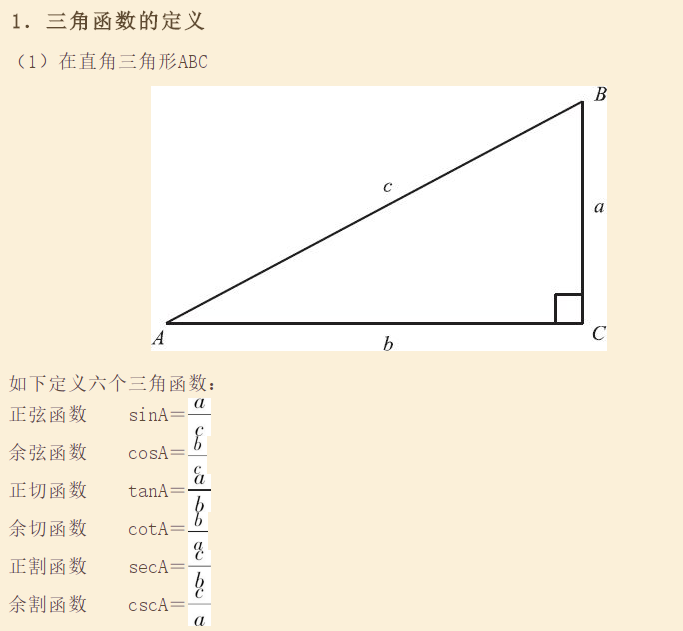二、诱导公式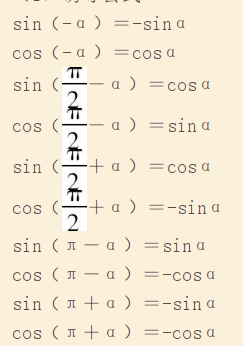三、倒数关系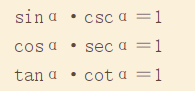四、平方关系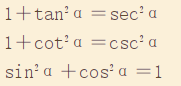五、两角和与差的三角函数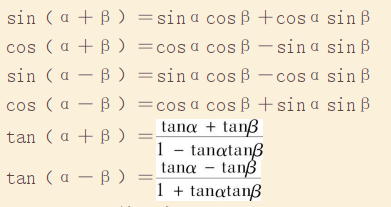六、积化和差公式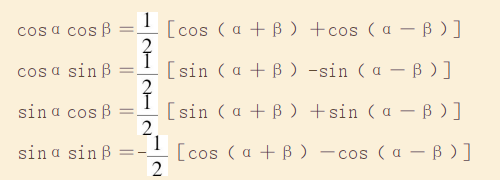七、和差化积公式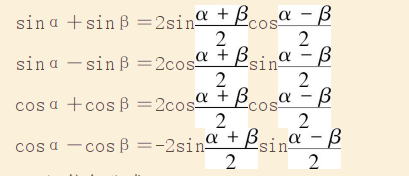八、倍角公式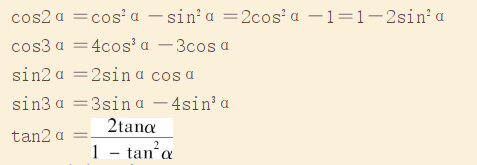九、半角公式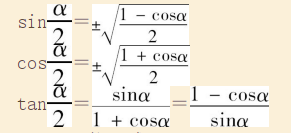十、万能公式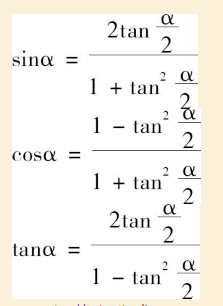十一、其他公式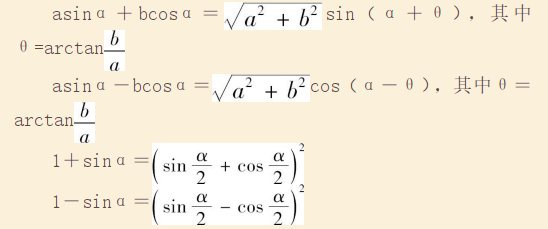展开全文• 目录：一、弧度制二、三角函数的基本定义三、同角三角函数基本关系四、三角函数的诱导公式五、三角函数的和差角公式六、倍角公式半角公式七、积化和差与和差化积公式八、万能公式九、辅助角公式十、求导十一、反...
• 高中三角函数公式大全：两角和公式、倍角公式、三倍角公式、半角公式 高中三角函数公式大全：和差化积、积化和差 高中三角函数公式大全：诱导公式、万能公式 高中三角函数公式大全：其他公式、其他非重点三角函数...高中数学 函数公式大全
• 转载自http://202.113.29.3/nankaisource/mathhands/Elementary%20mathematics/0103/010306/01030601.htm 1.倍角公式
• §4 三角函数的基本关系和诱导公式 1.同角三角函数的基本公式 2.同角三角函数换算表   x x x x ...
• 高中三角函数公式大全：两角和公式、倍角公式、三倍角公式、半角公式高中三角函数公式大全：和差化积、积化和差高中三角函数公式大全：诱导公式、万能公式高中三角函数公式大全：其他公式、其他非重点三角函数、双曲...
• sin cos tan cot sh ch th arsh arch 二倍角 三倍角 半角 两角和 诱导公式 万能公式 积化和差 和差化积
• 主要思路:从欧拉公式推证得四条积化和差公式,得到了三角函数中加减乘除的转换基础,之后的证明就非常简单了. 1我们首先从欧拉公式推出sinx和cosx 2再推出积化和差的四个基本公式 积化和差的具体推导只是一个非技巧...
• 温馨提示：内容较长，需耐心观看目录 一、定义二、同角三角函数 三、诱导公式 四、和差角公式 五、和差化积与积化和差公式 六、倍角公式半角公式 七、万能公式 八、辅助角公式 九、在三角函数中的恒等式与不等式 ...
• 三角函数总共由28个考点需要掌握，分别是：专题一：象限角及终边相同的角考点1：象限角的表示考点2：已知终边求角度考点3：半角平分法确定象限专题二：扇形的相关公式考点4：扇形的相关公式专题三：三角函数的定义...
• 1 基本三角公式定义： 诱导公式 基本关系 万能公式 重要基本公式  2倍角公式 半角公式 两角和差变形公式 和差化积 积化和差  ...
• §7 三角函数的有限和公式 *三角函数的有限和公式 §8 斜三角形基本定理、公式和解法 1.基本定理 *正弦定理    其中R为△ABC的外接圆半径 *余弦定理 注：...
• 三角函数定义 把角度θ作为自变量，在直角坐标系里画个半径为1的圆（单位圆），然后角的一边与X轴重合，顶点放在圆心，另一边...三角函数公式大全 两角和公式 倍角公式 三倍角公式 半角公式 和差化积 诱导公式 万能公式cos sin tan
• 基本公式： 倍角公式三角函数的幂： t=tan x/2代换： 加法公式三角函数的积： 半角公式计算机视觉
• ## 三角函数计算，Cordic 算法入门

万次阅读 多人点赞 2013-01-02 13:47:45
这种表格在人们刚刚产生三角函数的概念的时候就已经有了，它们通常是通过从已知值（比如sin(π/2)=1）开始并重复应用半角和和差公式而生成。 现在有了计算机，三角函数表便推出了历史的舞台。但是像我这样的喜欢...算法
• 包括倒数关系、商的关系、平方关系、诱导公式、两角和与差的三角函数公式、万能公式、半角的正弦、余弦和正切公式、三角函数的降幂公式、二倍角的正弦、余弦和正切公式、三倍角的正弦、余弦和正切公式、三角函数的和...
• 三角函数定义、三角函数线 三角函数特殊值 诱导公式 正弦函数与余弦函数的图像性质 正弦函数与余弦函数的平移拉伸 三角恒等变换：两角和与差的正弦、余弦、正切；半角、倍角公式；积化和差、和差化积公式；辅助角...
• 1、三角函数 2、两角和公式 3、二倍角公式 4、半角公式 5、和差化积 6、积化和差高等数学
• 1.诱导公式；2.两角和与差的三角函数；3.和差化积公式；4.积化和差公式；5.二倍角公式；6.半角公式；7.万能公式；8.其它公式(推导出来的 ) ；速查表。c